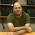## Monday, March 4, 2013

### Shrinkage in bimodal hierarchical models: Toward the modes, not the middle

In hierarchical models, the estimated values of intermediate-level parameters exhibit "shrinkage" because the higher-level distribution affects the intermediate-level parameter estimates. In typical applications, the form of the higher-level distribution is also estimated. And, in most typical applications, the higher-level distribution is unimodal, producing shrinkage of intermediate-level parameters is toward the middle of the higher-level distribution. Because this type of shrinkage is so prevalent, it is easy to think that shrinkage is always inward, toward the middle. But it does not have to be. This post shows a simple case of bimodal data producing a bimodal higher-level distribution, which causes shrinkage to be outward to the two modes. In other words, this post is a reminder that shrinkage is not toward the middle, shrinkage is toward the modes.

Consider a simple case of estimating the biases of several coins, and simultaneously estimating the distribution of biases across the coins. This is like estimating individual subject parameters (the coins) and group-level summary parameters (for the distribution across coins). For the jth coin, we observe a particular number of heads, Hj, out of its total number of flips, Nj. Denote the estimated bias of the coin as θj. Then the likelihood function is the usual product of Bernoulli's:
p(Hjj,Nj) = θjH (1-θj)(N-H)
The distribution of θj is here described by a beta distribution with shape parameters a and b:
p({θj}|a,b) = Πj dbeta(θj|a,b)
The overall likelihood of the parameters, for the particular data, is computed as the product of the equations above. Our goal is to find the parameter values, for {θj} and a and b, that maximize the likelihood.

In the two examples shown below there are 6 coins, each flipped 30 times. The proportion of flips that are heads is shown by the placement of the black dots in the figures below. For both examples, there are three coins that have fewer than 50% heads and three coins that have more than 50% heads. All that differs between the two examples is how extreme the separation is between the two clusters of coins.

In the first example, the two clusters of data are not separated by much. In the figure below, the black dots show the data. This first figure shows the likelihood if we choose values for θj that exactly match the observed proportion of heads in each coin, as shown by the blue circles, and we set a=1 and b=1 for the over-arching beta distribution:

We can find parameter values that produce a higher likelihood, however. In fact, the maximum likelihood estimates of the parameters are shown here:

Importantly, notice in the figure above that the blue circles, which represent the best estimates of the biases in the coins, are shrunken --- and toward the middle of the data.

But now consider what happens when the data are more extremely bimodal, as shown below. First, again, we consider choosing parameter values that match the proportions in the data and give a uniform higher-level distribution:

The maximum-likelihood estimates of the parameters are shown here:

Importantly, notice in the figure above that the blue circles, which represent the best estimates of the biases in the coins, are "shrunken" --- away from the middle of the data. The moral: Shrinkage is toward the modes of the higher-level distribution, not necessarily toward the middle.

#### 1 comment:

1.This is a very important point. In general our intuitions in models are informed by the linear model with Gaussian errors. Outside that environment many things can be quite different.